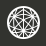Formatting Guide for Questions and Comments

For formatting mathematics, we use LaTeX throughout the site. This means that you can format the math parts of your question, comment or solution by putting certain code inside these brackets $$...$$. The tables below contain some symbols that you will need most often, with the "code" in the left column, and the result on the right.

Markdown codeAppears as
*italics* or _italics_ italics
**bold** or __bold__ bold
- bulleted
- list

*Include an additional line break (empty row) before the start of a list.
*Make sure there is a space between the hyphen and list item text.

• bulleted
• list
1. numbered
2. list
1. numbered
2. list
paragraph 1

paragraph 2

paragraph 1

paragraph 2

> This is a quote
This is a quote
![Brilliant Logo](http://cl.ly/image/3m1O2L2k380t)while True:
print "Solving problems now..."
print "All done."

while True:
print "Solving problems now..."
print "All done."
python
#By adding three tickmarks and a language name, I've added syntax highlighting.
while True:
print "Solving more problems..."
print "Achievement unlocked."

#By adding three tickmarks and a language name, I've added syntax highlighting.
while True:
print "Solving more problems..."
print "Achievement unlocked."
|| 1 || 2 || 3 || 4 ||
|| a || b || c || d ||
 1 2 3 4 a b c d
LaTeX CodeAppears as
1 + 2$1 + 2$
2 \times 3$2 \times 3$
2 \cdot 3$2 \cdot 3$
2 * 3$2 * 3$
2^3$2^3$
2^{34}$2^{34}$
2^34$2^34$
a_{i-1}$a_{i-1}$
a_i-1$a_i-1$
2^a_1$2^a_1$
\frac {2}{3}$\frac {2}{3}$
\frac 234$\frac 234$
LaTeX CodeAppears as
\frac {1}{1 + \frac {1}{x}}$\frac {1}{1 + \frac {1}{x}}$
\sqrt{2}$\sqrt{2}$
\sqrt{2}$\sqrt{2}$
1 \pm i$1 \pm i$
\frac{-b \pm \sqrt{b^2-4ac}}{2a}$\frac{-b \pm \sqrt{b^2-4ac}}{2a}$
2 < 3$2 < 3$
2 \leq 3$2 \leq 3$
3 \geq 2$3 \geq 2$
2 \neq 3$2 \neq 3$
|x|$|x|$
\boxed{123}$\boxed{123}$
LaTeX CodeAppears as
\infty$\infty$
1 + 2 + 3 + \ldots + 10$1 + 2 + 3 + \ldots + 10$
\sum_{i=1}^3$\sum_{i=1}^3$
\prod_{i=1}^{31}$\prod_{i=1}^{31}$
\displaystyle \sum_{i=1}^3$\displaystyle \sum_{i=1}^3$
\log_2 4^6$\log_2 4^6$
4 \equiv 2 \pmod{2}$4 \equiv 2 \pmod{2}$
{6 \choose 3}${6 \choose 3}$
\gcd(n,m)$\gcd(n,m)$
\angle ABC = 90 ^ \circ$\angle ABC = 90 ^ \circ$
LaTeX CodeAppears as
\sin \theta$\sin \theta$
\cos 90^\circ = 0$\cos 90^\circ = 0$
\tan \frac{\pi}{6} = \frac{1}{\sqrt{3}}$\tan \frac{\pi}{6} = \frac{1}{\sqrt{3}}$
\alpha, \beta, \gamma, \ldots$\alpha, \beta, \gamma, \ldots$
\overline{abba}$\overline{abba}$
\underline{abba}$\underline{abba}$
\rightarrow$\rightarrow$
\Rightarrow$\Rightarrow$

For a more extensive list of LaTeX symbols, you may refer to this Wikipedia page.

LaTeX CodeAppears as
\ce{H2O}$\ce{H2O}$
\ce{CrO4^2-}$\ce{CrO4^2-}$
\ce{A <--> B}$\ce{A <--> B}$
\ce{A ->[x_i] B}$\ce{A ->[x_i] B}$
\ce{CO2 + C -> 2 CO}$\ce{CO2 + C -> 2 CO}$
\ce{A\bond{~--}B\bond{~=}C\bond{-~-}D}$\ce{A\bond{~--}B\bond{~=}C\bond{-~-}D}$
×